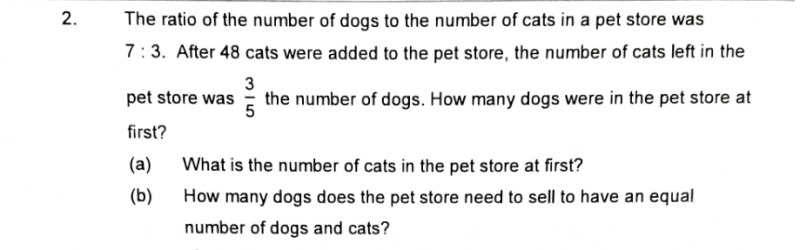# QuestionDear Parents, please assist on this question. Tq.

Dogs                            Cats

Before            7u x 3=21u                3u x5 = 15u

Add cats                                          +48 x 5 = 240

After               5px3 = 15p                 3px 5 = 15p

So 21u = 15u + 240

6u = 240

u = 40

Number of dogs in pet store at first = 7×40 = 280

(a) Number of cats in pet store at first = 3×40 = 120

(b) Number of cats after adding 48 = 120+48 = 168

To have equal number of dogs to cats in the store, the store must sell 280-168 = 112 dogs

0 Replies 1 Like